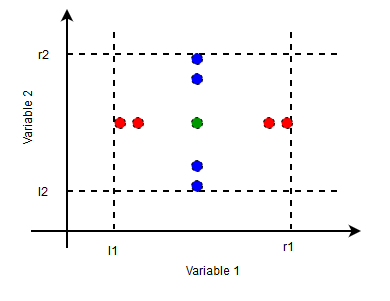# Boundary Value Analysis – Triangle Problem

Boundary Value Analysis (BVA) is a black box software testing technique where test cases are designed using boundary values. BVA is based on the single fault assumption, also known as critical fault assumption which states that failures are rarely the product of two or more simultaneous faults. Hence while designing the test cases for BVA we keep all but one variable to the nominal value and allowing the remaining variable to take the extreme value.

Test Case Design for BVA:
While designing the test cases for BVA first we determine the number of input variables in the problem. For each input variable, we then determine the range of values it can take. Then we determine the extreme values and nominal value for each input variable.

Consider an input variable t taking values in the range [l, r].Extreme values for t are –```t = l
t = l+1
t = r-1
t = r ```

The nominal value for the variable can be any value in the range (l, r).
In most of the BVA implementations, it is taken as the middle value of the range (r+l)/2.
The figure on the right shows the nominal and extreme values for boundary value of analysis of a two variable problem.

Under the single fault assumption, the total number of test cases in BVA for a problem with n inputs is 4n+1.
The 4n cases correspond to the test cases with the four extreme values of each variable keeping the other n-1 variable at nominal value. The one additional case is where all variables are held at a nominal value.

One of the common problem for Test Case Design using BVA is the Triangle Problem that is discussed below –

Triangle Problem accepts three integers – a, b, c as three sides of the triangle .We also define a range for the sides of the triangle as [l, r] where l>0. It returns the type of triangle (Scalene, Isosceles, Equilateral, Not a Triangle) formed by a, b, c.

For a, b, c to form a triangle the following conditions should be satisfied –

```a < b+c
b < a+c
c < a+b ```

If any of these conditions is violated output is Not a Triangle.

• For Equilateral Triangle all the sides are equal.
• For Isosceles Triangle exactly one pair of sides is equal.
• For Scalene Triangle all the sides are different.

The table shows the Test Cases Design for the Triangle Problem.The range value [l, r] is taken as [1, 100] and nominal value is taken as 50.
The total test cases is,

`4n+1 = 4*3+1 = 13 `

Test Case ID a b c Expected Output
T1 1 50 50 Isosceles
T2 2 50 50 Isosceles
T3 99 50 50 Isosceles
T4 100 50 50 Not a Traingle
T5 50 50 50 Equilateral
T6 50 1 50 Isosceles
T7 50 2 50 Isosceles
T8 50 99 50 Isosceles
T9 50 100 50 Not a Triangle
T10 50 50 1 Isosceles
T11 50 50 2 Isosceles
T12 50 50 99 Isosceles
T13 50 50 100 Not a Triangle

GeeksforGeeks has prepared a complete interview preparation course with premium videos, theory, practice problems, TA support and many more features. Please refer Placement 100 for details

My Personal Notes arrow_drop_upCheck out this Author's contributed articles.

If you like GeeksforGeeks and would like to contribute, you can also write an article using contribute.geeksforgeeks.org or mail your article to contribute@geeksforgeeks.org. See your article appearing on the GeeksforGeeks main page and help other Geeks.

Please Improve this article if you find anything incorrect by clicking on the "Improve Article" button below.

Article Tags :

Be the First to upvote.

Please write to us at contribute@geeksforgeeks.org to report any issue with the above content.